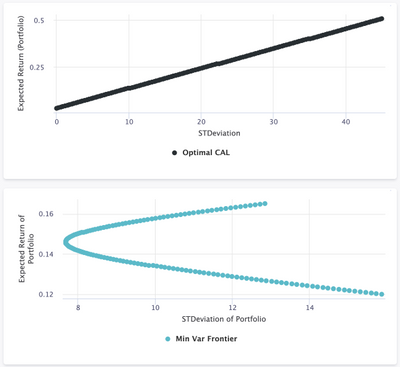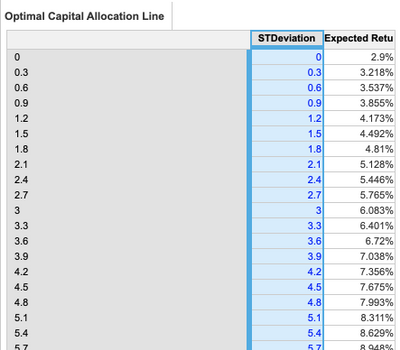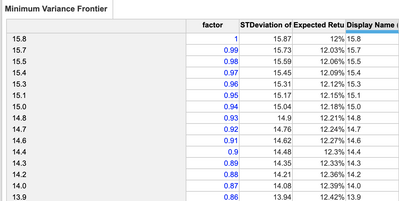# Multi-Dimensional Scatter Plot Alternatives?Hi all,

Bit of a complex one here. The application use case is calculating the Minimum Variance Efficient Portfolio for those familiar with Finance / Portfolio Management.

The Anaplan question to be solved in simple terms, is how can I combine these two charts to display as two different "lines" within the same chart (in other words, within the same module). The issue is that in theory, both axes  would be a logarithmic scale, since for each incremental risk factor, you would have a unique expected return...Info on the above screenshot:

Optimal Capital Allocation Line (CAL) is the only dimension in the related module, where each data plot is calculating the expected return an investor must be compensated with for each incremental risk unit (in this case, standard deviation, or Beta, of a security)... That module looks like this:The second graph, displaying the Minimum variance frontier, is also currently dimensioned by one list called the Minimum Variance Frontier. This plots the standard deviation and expected return of a two-security portfolio based on their correlation. The calculation achieves, in simple terms, the curve of your return vs. risk trade off based on your two selected securities. That module looks like this:How can I display these two data sets plotted against each other? The only idea I can come up with at the moment, that I have not proven to work after many tries is:

Utilizing a dot chart - In a new module, make Standard Deviation and Expected Return as two separate lists. Make "Optimal CAL" and "Min Var Frontier" the line items, and write formulas that apply a data point where the intersection of your standard deviation and your expected return are true based on which line item you are calculating (these would be two different formulas of course).

Appreciate any insight!

Happy building.

Tagged: